• We know by now that PCA can reduce the data while representing original data, thus making faster learning algorithm.
• Still, choose where to apply PCA wisely, not all area can be benefit from PCA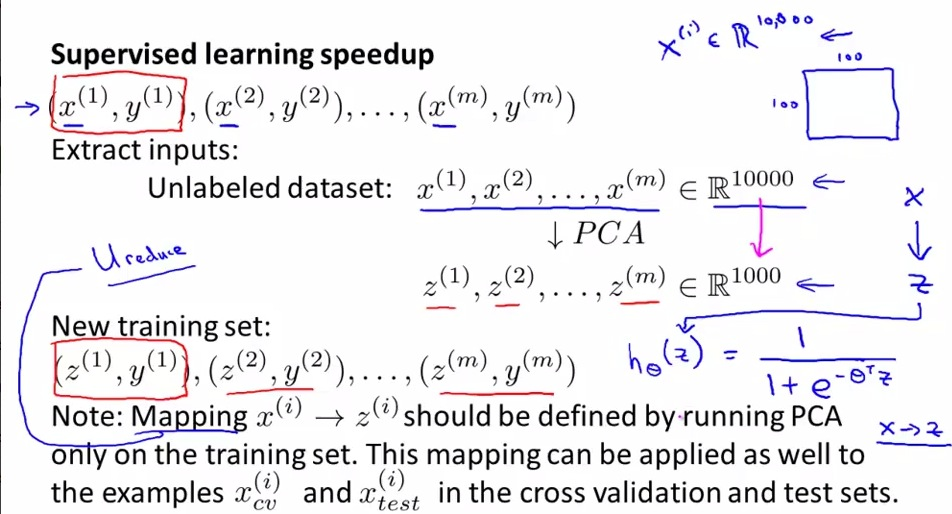• We can use PCA for speedup our supervised learning algorithm.
• For this particular example, let's say we want to reduce the dimension of the data, down to one tenth of the original
• Extract only x-value from the original, so it become unlabeled dataset
• Then apply  the new x-index(z-value) to coressponding y-value so it become the new training set
• Replace x with z, and run the hyphothesis.
• As warning above, use PCA Ureduce only for training set. Then use same mapping to cv and test set.
• For many problem max is 1/10 for keeping most retain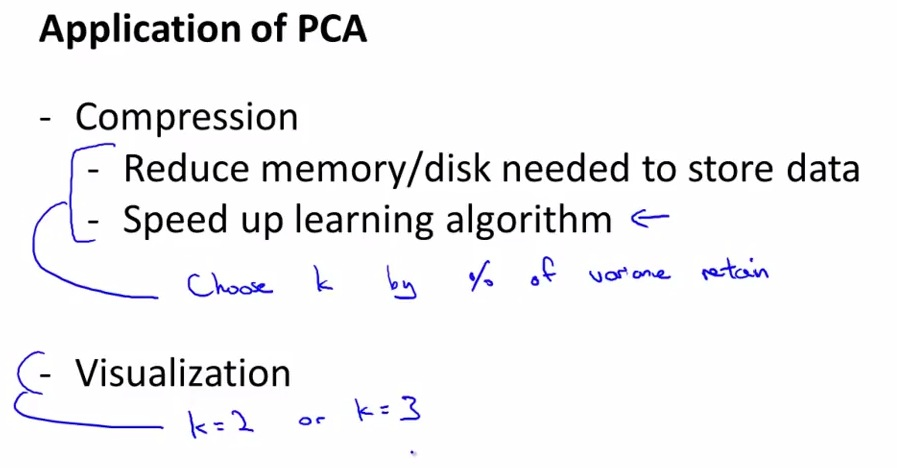• Choose k wisely
• only use k = 2/3 for visualization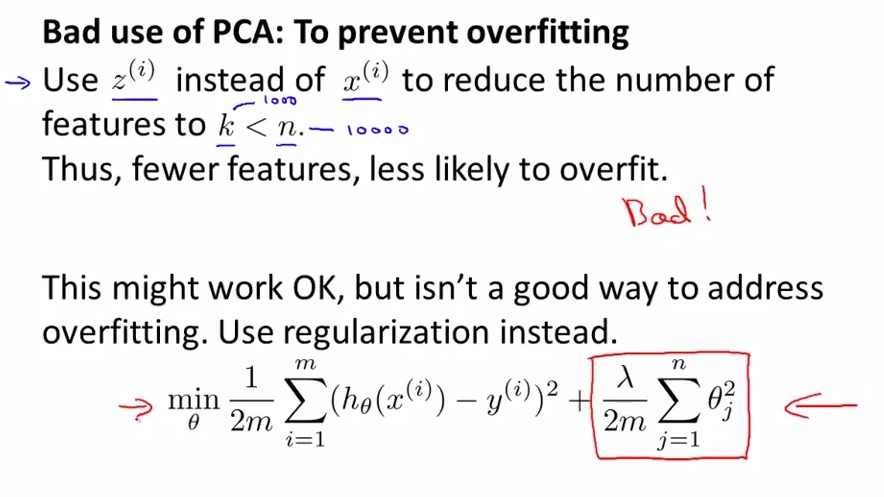• Here we see how we misuse PCA for overfitting, for solving overfitting problem.
• y-value doesn't incorporated  for PCA to take into account.
• Thus give a bad compression for representing original data that should take y-value as an input, resulting in throwing away some valuable information
• Regularization would works just fine, less likely throw away some valuable information for logistic regression or neural networks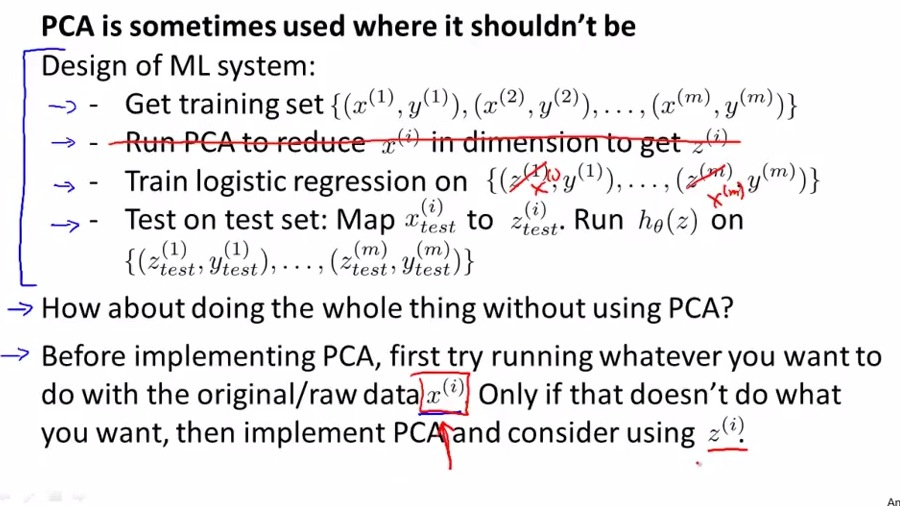• Adding PCA is a more complicated
• If data too large, or anything else, ex doesn't work. then use PCA. But not recommended to use PCA as a basic plan/first plan. Only if things doesn't work. PCA is a little complicated that shouldn't be at first plan for reducing the data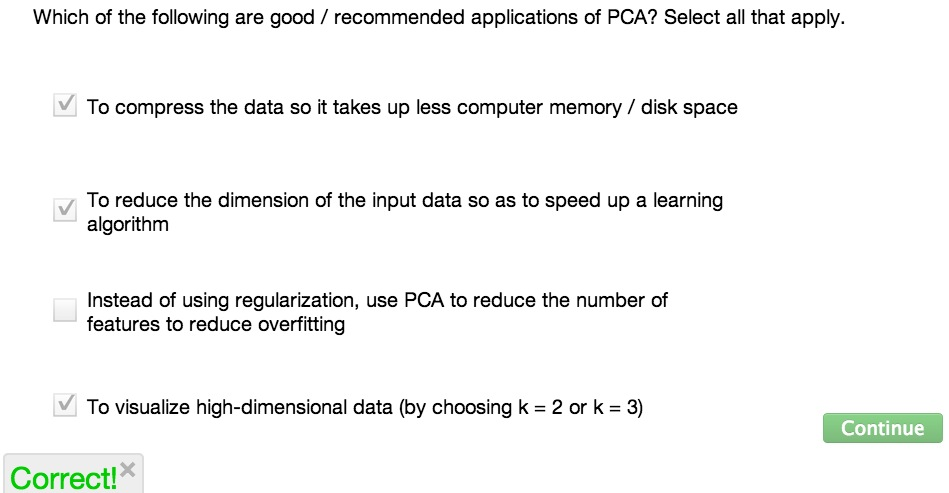• PCA is really benefit for appropriate application
• Use PCA for compression,reducing memory usage, visualization
• PCA should be implemented wisely